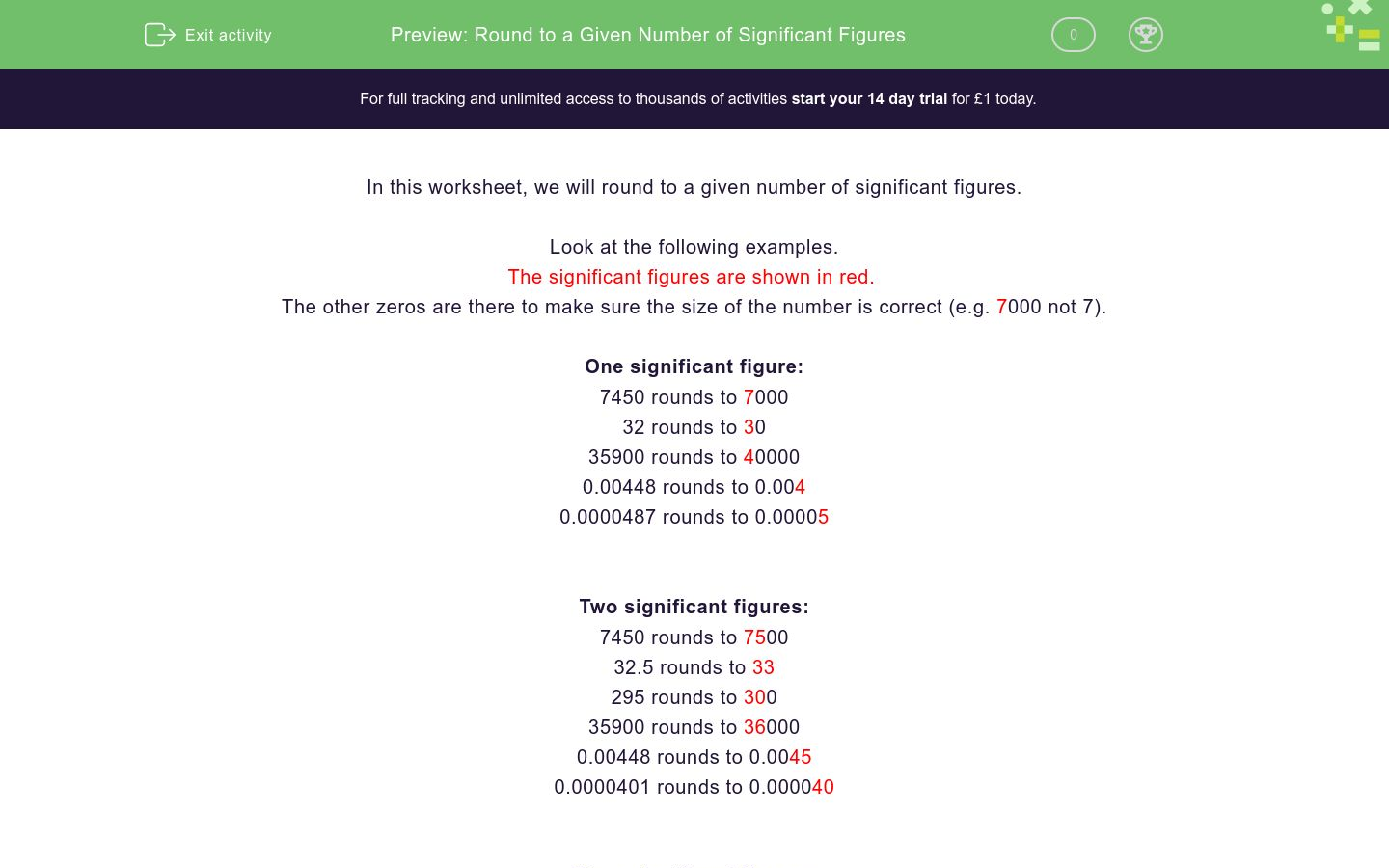# Round to a Given Number of Significant Figures

In this worksheet, students round numbers to a given number of significant figures.Key stage:  KS 4

Curriculum topic:  Number

Curriculum subtopic:  Apply/Interpret Limits of Accuracy When Rounding/Truncating

Difficulty level:### QUESTION 1 of 10

This worksheet is about rounding to a given number of significant figures.

Look at the following examples.

The significant figures are shown in red.  The other zeros are there to make sure the size of the number is correct (e.g. 7000 not 7)

One significant figure

7450 rounds to 7000

32 rounds to 30

35900 rounds to 40000

0.00448 rounds to 0.004

0.0000487 rounds to 0.00005

Two significant figures

7450 rounds to 7500

32.5 rounds to 33

295 rounds to 300

35900 rounds to 36000

0.00448 rounds to 0.0045

0.0000401 rounds to 0.000040

Three significant figures

7450 rounds to 7450

32.99 rounds to 33.0

35910 rounds to 35900

35990 rounds to 36000

0.004487 rounds to 0.00449

0.00004895 rounds to 0.0000490

Round the following number to 1 significant figure.

275

Round the following number to 1 significant figure.

0.06524

Round the following number to 1 significant figure.

164

Round the following number to 1 significant figure.

0.024562

Round the following number to 2 significant figures.

0.02572

Round the following number to 2 significant figures.

1244

Round the following number to 2 significant figures.

0.05254

Round the following number to 2 significant figures.

0.0005478

Round the following number to 3 significant figures.

83659

Round the following number to 3 significant figures.

0.0073568

• Question 1

Round the following number to 1 significant figure.

275

CORRECT ANSWER
300
EDDIE SAYS
275 rounds up to 300.
• Question 2

Round the following number to 1 significant figure.

0.06524

CORRECT ANSWER
0.07
EDDIE SAYS
0.065... rounds up to 0.07.
• Question 3

Round the following number to 1 significant figure.

164

CORRECT ANSWER
200
EDDIE SAYS
164 rounds up to 200, because it is closer to 200 than 100.
• Question 4

Round the following number to 1 significant figure.

0.024562

CORRECT ANSWER
0.02
EDDIE SAYS
0.024562 rounds down to 0.02, because the next significant figure - 4 - is less than the number 5.
• Question 5

Round the following number to 2 significant figures.

0.02572

CORRECT ANSWER
0.026
EDDIE SAYS
Here, we take into consideration the number 7, which is the 3rd significant figure. 7 is above 5, which means that we round the number UP to 0.026.
• Question 6

Round the following number to 2 significant figures.

1244

CORRECT ANSWER
1200
EDDIE SAYS
1244 is closer to 1200 than 1300, so we round down.
• Question 7

Round the following number to 2 significant figures.

0.05254

CORRECT ANSWER
0.053
EDDIE SAYS
Here, we round up, because the 3rd significant figure is 5. So, the answer is 0.053.
• Question 8

Round the following number to 2 significant figures.

0.0005478

CORRECT ANSWER
0.00055
EDDIE SAYS
Again, we have rounded up because the number after the 2nd significant figure is higher than five.
• Question 9

Round the following number to 3 significant figures.

83659

CORRECT ANSWER
83700
EDDIE SAYS
Again, we round up here because 83659 is closer to 83700 than 83600.
• Question 10

Round the following number to 3 significant figures.

0.0073568

CORRECT ANSWER
0.00736
EDDIE SAYS
The answer here is 0.00736, because the 4th significant figure is higher than five.
---- OR ----

Sign up for a £1 trial so you can track and measure your child's progress on this activity.

### What is EdPlace?

We're your National Curriculum aligned online education content provider helping each child succeed in English, maths and science from year 1 to GCSE. With an EdPlace account you’ll be able to track and measure progress, helping each child achieve their best. We build confidence and attainment by personalising each child’s learning at a level that suits them.

Start your £1 trial